## Example Questions

### Example Question #1 : How To Find The Angle Of Clock Hands

If is 2:00 PM, what is the measure of the angle between the minute and hour hands of the clock?

45 degrees

120 degrees

60 degrees

90 degrees

30 degrees

60 degrees

Explanation:

First note that a clock is a circle made of 360 degrees, and that each number represents an angle and the separation between them is 360/12 = 30. And at 2:00, the minute hand is on the 12 and the hour hand is on the 2.  The correct answer is 2 * 30 = 60 degrees.

### Example Question #2 : How To Find The Angle Of Clock Hands

It is 4 o’clock.  What is the measure of the angle formed between the hour hand and the minute hand?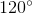Explanation:

At four o’clock the minute hand is on the 12 and the hour hand is on the 4.  The angle formed is 4/12 of the total number of degrees in a circle, 360.

4/12 * 360 = 120 degrees

### Example Question #3 : How To Find The Angle Of Clock Hands

If a clock reads 8:15 PM, what angle do the hands make?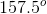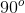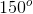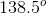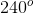Explanation:

A clock is a circle, and a circle always contains 360 degrees. Since there are 60 minutes on a clock, each minute mark is 6 degrees.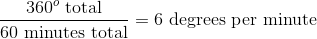The minute hand on the clock will point at 15 minutes, allowing us to calculate it's position on the circle.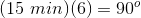Since there are 12 hours on the clock, each hour mark is 30 degrees.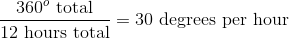We can calculate where the hour hand will be at 8:00.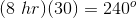However, the hour hand will actually be between the 8 and the 9, since we are looking at 8:15 rather than an absolute hour mark. 15 minutes is equal to one-fourth of an hour. Use the same equation to find the additional position of the hour hand.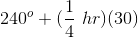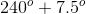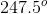We are looking for the angle between the two hands of the clock. The will be equal to the difference between the two angle measures.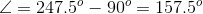### Example Question #1 : How To Find The Angle Of Clock Hands

What is the measure of the smaller angle formed by the hands of an analog watch if the hour hand is on the 10 and the minute hand is on the 2?

56°

45°

90°

30°

120°

120°

Explanation:

A analog clock is divided up into 12 sectors, based on the numbers 1–12. One sector represents 30 degrees (360/12 = 30). If the hour hand is directly on the 10, and the minute hand is on the 2, that means there are 4 sectors of 30 degrees between then, thus they are 120 degrees apart (30 * 4 = 120).

### Example Question #5 : How To Find The Angle Of Clock Hands

At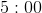, what angle is between the hour and minute hand on a clock?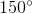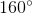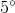Explanation:

At, the hour hand is on theand the minute hand is at the. There arespaces on a clock, and these hands are separated byspaces.

Thus, the angle between them is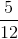the degrees of the entire clcok, which is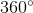.

Therefore, we multiply these to get our answer.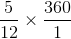We can cancel out as we multiply to get: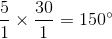### Example Question #1 : How To Find The Angle Of Clock Hands

What is the measure, in degrees, of the acute angle formed by the hands of a 12-hour clock that reads exactly 3:10?

72°

65°

35°

55°

60°

35°

Explanation:

The entire clock measures 360°. As the clock is divided into 12 sections, the distance between each number is equivalent to 30° (360/12). The distance between the 2 and the 3 on the clock is 30°.  One has to account, however, for the 10 minutes that have passed. 10 minutes is 1/6 of an hour so the hour hand has also moved 1/6 of the distance between the 3 and the 4, which adds 5° (1/6 of 30°). The total measure of the angle, therefore, is 35°.

### Example Question #7 : How To Find The Angle Of Clock Hands

If it is 4:00, what is the measure of the angle between the minute and hour hands of the clock?

90 degrees

45 degrees

120 degrees

100 degrees

125 degrees

120 degrees

Explanation:

A clock takes the shape of a circle, which is composed of 360 degrees. There are 12 numbers on a clock that represent the hours. With this in mind, we can say that each number represents an angle. The measure of the angle between each number is given by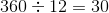.

If it is 4:00, then the minute hand is pointing towards 12 while the hour hand points towards 4.

Therefore, we can say that the angle between the two hands is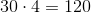degrees.

Another way to think of this is to imagine the clock at a nearby time. At 3:00, the hands of the clock form a right angle of 90 degrees. Since we know that each number on the clock is separated by 30 degrees, we can simply add 30 to 90 degrees and get 120 degrees for the angle at 4:00.

### All SAT Math Resources